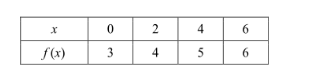4008-125-888

# 基础代数：线性方程 - 直线的位置关系（上）

2017年05月02日 02:16来源：互联网作者：上海管理员

线性函数的表现形式：

The general form of the equation is

The slope-intercept form is

The slope of the line is represented by m and is defined to be the ratio of, where and are any two points on the line. The y-intercept is b.

The slope-point form is直线的位置关系：

If the slopes if line l and k are each defined (that is, if neither line is a vertical line), then

(1)line l and k are parallel if and only if they have the same slope;

(2)Line l and line k are perpendicular if and only if the product of their slopes is -1.

例题9. f(x) = 2x – 1 and g(x) is a linear function that is perpendicular to f(x). If (0, 4) is a point of g(x), at what point do f(x) and g(x) intersect?

(A) (0, -1)

(B) (1, 1)

(C) (2, 3)

(D) (3, 2)

Solution：

g(x)的斜率为，过(0,4)点，所以直线方程为：

两个方程联立，解出 x=2, y=3。

答案：C。

例题6. Luca’s pays \$1195 per month for rent plus 10 cents per kilowatt hour (kWh) used for electricity. If Luca used x kWh in one month, which expression best represents the amount of money in dollars Luca needs to pay for his apartment?

(A) 1195 + 0.1x

(B) (1195 + 0.1)x

(C) 1195 + 10x

(D) (1195 + 1)x

Solution：

1195为基础费用，10cents是每kWh的增加费用，所以总费用的方程为：

答案：A.

例题7. Which of the following equations has the same slope as 2y + 6x = 5?

(A) x + 3y = 1

(B) 3x = –y + 5

(C) y – 3x = 4

(D) 6y = 2x – 1

Solution:

2y + 6x = 5是直线方程的一般形式，改写为斜截式：

，斜率为-3。再从选项中寻找斜率同样为-3的直线。

答案：B。

例题6. If f(x) = 2x + 2 is a linear function, which of the following is true for 4f(x)?

(A) The slope is four times steeper than f(x).

(B) The slope is four times less steep than f(x).

(C) All values of x are four times greater than f(x) for the same values of y.

(D) The slope changes, but the y-intercept remains the same as f(x).

Solution：

4f(x)的图像是将y轴拉伸4倍，4f(x)=8x+8。图像与X轴的交点不变，与Y轴的交点扩大4倍，斜率也增加4倍。

答案：A。例题2. Which of the following expressions defines f(x) in the table above?

(A) f(x) = x + 3

(B) f(x) = x + 3

(C) f(x) = x

(D) f(x) = 2x

Solution:

首先判断斜率：，再代入一个点计算，答案为B。

SAT香港考试团## 热门活动

#### 相关内容

1、如转载本网原创文章，请表明出处;
2、本网转载媒体稿件旨在传播更多有益信息，并不代表同意该观点，本网不承担稿件侵权行为的连带责任；
3、如本网转载稿、资料分享涉及版权等问题，请作者见稿后速与新航道联系（电话：021-64380066），我们会第一时间删除。

-